# All Classes and Courses

## Find the resources you need to learn about computational fields and further your understanding of Wolfram technologies.

Click any button to browse all catalog resources in a particular area or use the filters to refine your search. The catalog includes free, self-paced, interactive courses, standalone video lessons, video courses and scheduled instructor-led courses as well as archived and special events. Learn about the different course types.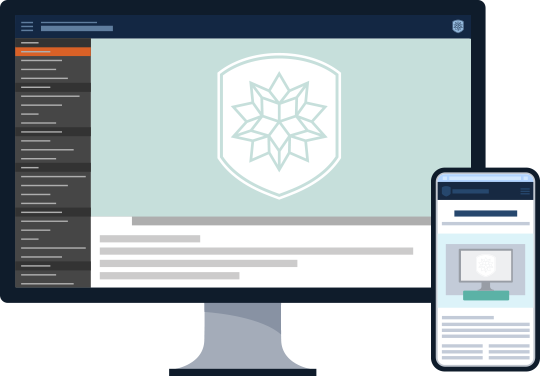Refine & Sort Showing filtered results
• Course Type
• Difficulty
• Certification
• Time to Complete
• Clear options
• Sort by
• Clear options

42 results

##### Calculus Concepts and Applications
###### Video Course | FREE

This video course begins with an overview of basic calculus operations and takes you on an exploration of Wolfram Language functions, including those used for computing integrals and solving differential equations. Learn from one of our symbolic computing experts about applications of calculus to real-world problems, gain insight into the technology underlying our built-in calculus functions, and find some interesting historical mathematical references as well as resources for further study. Allow the Wolfram Language to introduce you to—or help you to rediscover—some of the beauty and utility of calculus.

###### Course Overview
• Video 130 minutes
• Video 223 minutes
##### Daily Study Group: Introduction to Discrete Mathematics
###### Special Event | FREE

Certification Levels: CompletionLevel 1

This Daily Study Group follows lessons from the upcoming Introduction to Discrete Mathematics course on Wolfram U. The course explores the main branches of discrete mathematics and simple applications in computer science. It follows and expands on topics covered in discrete mathematics or mathematics of computer science courses.

##### Daily Study Group: Introduction to Multivariable Calculus
###### Special Event | FREE

Certification Levels: CompletionLevel 1

This Daily Study Group follows lessons from the upcoming Wolfram U comprehensive introduction to multivariable calculus course. The course extends concepts from single-variable calculus to multiple dimensions. Multivariable calculus follows and expands on topics covered in AP Calculus BC courses.

##### Discrete Calculus with Wolfram Language
###### Video Course | FREE

This course discusses the basics, history and real-world applications of discrete calculus as well as associated Wolfram Language functionality.

###### Course Overview
• Video 15 minutes
• Video 210 minutes
• Video 39 minutes
• Video 49 minutes
• Video 512 minutes
• Video 611 minutes
• Video 712 minutes
• Video 811 minutes
• Video 912 minutes
• Video 1019 minutes
• Video 1120 minutes
• Video 1213 minutes
• Video 1317 minutes
• Video 148 minutes
• Video 1513 minutes
##### Introduction to Calculus
###### Interactive Course | FREE

Requirements: This course requires no prior knowledge of Mathematica or the Wolfram Language. Prerequisites for calculus include Algebra I (elementary algebra), Algebra II (intermediate algebra), elementary geometry and trigonometry or precalculus.

Certification Levels: CompletionLevel 1

A comprehensive introduction to fundamental concepts in calculus, including video lessons and interactive notebooks. Follow along with the examples in the Wolfram Cloud and use the material to prepare for the AP Calculus AB exam. The course starts with functions and limits, followed by differential calculus and its applications, and then moves on to integral calculus and its applications. Problem sessions, exercises, quizzes and a sample exam are provided for self-paced assessment. Earn a certificate by watching all lesson and problem session videos and completing the quizzes with a passing grade.

###### Course Overview
• Section 185 minutes
• Section 252 minutes
• Section 355 minutes
• Section 450 minutes
• Section 579 minutes
• Section 656 minutes
• Section 748 minutes
• Section 835 minutes
• Section 944 minutes
• Section 1042 minutes
• Section 1138 minutes
##### Introduction to Cryptography
###### Interactive Course | FREE

Requirements: This course requires a basic understanding of number theory, algorithms, discrete mathematics and modular arithmetic and basic-level skills in any programming language.

Certification Levels: Completion

This course gives an introduction to the concepts, underlying math, principles and techniques of historic and modern cryptography; ties cryptographic protocols to real-world scenarios; and gives a necessary understanding of issues related to information security in general.

###### Course Overview
• Section 122 minutes
• Section 213 minutes
• Section 326 minutes
• Section 416 minutes
• Section 545 minutes
• Section 650 minutes
• Section 745 minutes
• Section 816 minutes
##### Introduction to Differential Equations
###### Interactive Course | FREE

Requirements: This course requires no prior knowledge of Mathematica or the Wolfram Language. Prerequisites for differential equations include calculus and linear algebra.

Certification Levels: CompletionLevel 1

A comprehensive introduction to fundamental concepts and solution methods for differential equations, including video lessons and interactive notebooks. Follow along with the examples in the Wolfram Cloud and use the material to prepare for courses in natural science, engineering, economics and other fields. The course starts with a discussion of direction fields and methods for solving first-order differential equations, followed by the study of second-order equations and their applications, and then moves on to solving systems of differential equations. Problem sessions, exercises and quizzes are provided for self-paced assessment. Earn a certificate by watching all lesson and problem session videos and completing the quizzes with a passing grade. Level I certification in Differential Equations is awarded to those who meet the completion requirements and also pass the course final exam.

###### Course Overview
• Section 156 minutes
• Section 241 minutes
• Section 351 minutes
• Section 443 minutes
• Section 570 minutes
• Section 641 minutes
• Section 734 minutes
• Section 866 minutes
##### Introduction to Elementary Algebra
###### Interactive Course | FREE

Certification Levels: CompletionLevel 1

Elementary algebra is a prerequisite for multiple mathematics and statistics courses, and a strong foundation in algebra can help you better understand more advanced math. This course introduces the basic concepts of algebra as well as common types of problems and how to solve them. Start with algebraic definitions and then move on to linear equations, linear inequalities, polynomials and quadratic equations. The instructor also discusses word problem examples in each section and walks through the steps to finding a solution.

###### Course Overview
• Section 179 minutes
• Section 289 minutes
• Section 379 minutes
• Section 466 minutes
• Section 565 minutes
• Section 679 minutes
• Section 715 minutes
##### Introduction to Fractional Calculus
###### Video Course | FREE

Learn about computing fractional derivatives and using the popular Laplace transform technique to solve systems of linear fractional differential equations using Wolfram Language. The first video describes the basics of fractional calculus, defines some of the common differintegrals and introduces the built-in FractionalD and CaputoD functions. The second video focuses on using LaplaceTransform and InverseLaplaceTransform to convert from time domain to frequency domain and back again. It also demonstrates how you can combine the Laplace transform with MittagLefflerE functions and CaputoD derivatives. The final video provides more background on fractional calculus and its uses and showcases demonstrative examples of both single fractional differential equations and systems of linear fractional differential equations.

###### Course Overview
• Video 118 minutes
• Video 214 minutes
• Video 317 minutes
##### Introduction to Linear Algebra
###### Interactive Course | FREE

Requirements: This course requires no prior knowledge of Mathematica or the Wolfram Language. Prerequisites for linear algebra include algebra, elementary geometry and trigonometry.

Certification Levels: CompletionLevel 1

A comprehensive introduction to fundamental concepts in linear algebra, including video lessons and interactive notebooks. Follow along with the examples in the Wolfram Cloud and use the material to prepare for courses in data science, engineering and other fields. The course starts with linear equations and matrices, followed by determinants and eigenvalues, and then moves on to inner products and the singular value decomposition. Application sessions are included to show uses of linear algebra in the real world. Exercises and quizzes are provided for self-paced assessment.

###### Course Overview
• Section 138 minutes
• Section 241 minutes
• Section 336 minutes
• Section 426 minutes
• Section 536 minutes
##### Introduction to Mathematica for Students and Teachers
###### Video Course | FREE

Certification Levels: Level 1

This video course provides an introduction to Mathematica for students, teachers and beginners of all kinds. It quickly covers a wide range of topics from algebra to calculus using examples, all delivered from the viewpoint of a highly skilled and experienced classroom teacher. It starts with the concept of a computational notebook and builds step by step to more advanced topics such as plotting, graphics and solving equations. The course includes techniques for entering mathematical notation using your keyboard and palette buttons and finding help using the Wolfram Documentation Center. The course is recorded by Ruth Dover, a math instructor and Wolfram Innovator who has taught thousands of students how to use Mathematica and Wolfram Language over the course of her career.

###### Course Overview
• Video 116 minutes
• Video 29 minutes
• Video 313 minutes
• Video 411 minutes
• Video 515 minutes
• Video 618 minutes
• Video 711 minutes
• Video 87 minutes
• Video 914 minutes
• Section 1013 minutes
• Section 1124 minutes
• Video 1220 minutes
• Video 1313 minutes
• Video 1411 minutes
• Video 1518 minutes
##### Introduction to Probability
###### Interactive Course | FREE

Requirements: This course requires basic working knowledge of Mathematica or Wolfram Language. Prerequisites for probability include Algebra I (elementary algebra) and Algebra II (intermediate algebra).

Certification Levels: CompletionLevel 1

Probability is the branch of mathematics that deals with random phenomena. Using real-world examples, this comprehensive introduction to probability and statistics can help prepare you for coursework and research in natural science, engineering, finance, medicine, data science and other fields. The course starts with a discussion of basic probability concepts, followed by the study of random variables as well as common probability distributions and their applications. These topics are typically found in basic probability courses and serve as great preparation for statistics, data science and the professional examination Exam P from the Society of Actuaries.

###### Course Overview
• Section 151 minutes
• Section 239 minutes
• Section 332 minutes
• Section 434 minutes
• Section 527 minutes
• Section 626 minutes
##### Introduction to Statistics
###### Interactive Course | FREE

Requirements: This course requires basic working knowledge of Mathematica or Wolfram Language. Prerequisites for statistics include Algebra I (elementary algebra) and Algebra II (intermediate algebra).

Certification Levels: CompletionLevel 1

Statistics is the branch of mathematics concerned with collecting, describing, analyzing and interpreting data and trends in science, industry and society. This course introduces techniques for data visualization and descriptive statistics, methods for calculating confidence intervals and tools for hypothesis testing. Practical examples are used to demonstrate the application of statistical methods. The topics in this course are typically found in entry-level college statistics courses and serve as great preparation for the AP Statistics exam.

###### Course Overview
• Section 112 minutes
• Section 210 minutes
• Section 311 minutes
• Section 410 minutes
• Section 512 minutes
• Section 617 minutes
• Section 77 minutes
• Section 812 minutes
• Section 99 minutes
• Section 1012 minutes
• Section 1117 minutes
• Section 1215 minutes
• Section 1318 minutes
• Section 1410 minutes
• Section 1510 minutes
##### Introduction to the Wolfram Quantum Computation Framework (Study Group Sessions)
###### Archived Event | FREE

Follow these Daily Study Group sessions for an introduction to the Wolfram Quantum Computation Framework and how to use it in quantum computing. The foundation of the framework is a high-level symbolic expression representation of gates, unitaries, measurements, CPTP channels and so on. The framework is intuitive and flexible enough to model higher-order processes, such as quantum switches, using the several named states and operators built into the framework. Topics include basic concepts of quantum computation, including quantum states, transformations, algorithms, operators, gates, circuits and how to use Wolfram Language to run them on quantum hardware. You will learn how to simulate a quantum computer that operates based on the laws of quantum mechanics with Wolfram Language.

###### Course Overview
• Video 163 minutes
• Video 276 minutes
• Video 372 minutes
• Video 476 minutes
• Video 586 minutes
##### New in Wolfram Language 13.3 Webinar Series
###### Archived Event | FREE

There's a lot that's new in Version 13.3 of Wolfram Language and Mathematica, including a built-in subsystem around LLMs. Since the launch 35 years ago of Version 1.0, the long-term objective at Wolfram has always been to build a full-scale computational language that can represent everything computationally, in a way that’s effective for both computers and humans. This three-part webinar series features Chat Notebooks, LLMFunctions, math milestones, AR-VR publishing, interactive visualizations and system models for real-world data. Our experts show you ways in which our language has become a unique bridge between humans, AIs and computation.

###### Course Overview
• Video 177 minutes
• Video 253 minutes
• Video 366 minutes
##### Signals, Systems and Signal Processing
###### Interactive Course | FREE

Requirements: This course requires basic working knowledge of the Wolfram Language.

Certification Levels: Completion

This course gives an introduction to the concepts, mathematics, principles and techniques of signal processing in linear, time-invariant (LTI) systems. The course covers methods of analysis for both continuous-time and discrete-time signals and systems, presents sampling and gives an elementary introduction to filter design. Many everyday signal processing examples are included. The concepts and methods of signals and systems presented here play an important role in many areas of science and engineering, and therefore the course should be of interest to a broad range of students.

###### Course Overview
• Section 19 minutes
• Section 251 minutes
• Section 342 minutes
• Section 440 minutes
• Section 544 minutes
• Section 642 minutes
• Section 744 minutes
• Section 836 minutes
• Section 928 minutes

A Guide to Mathematics with Wolfram Language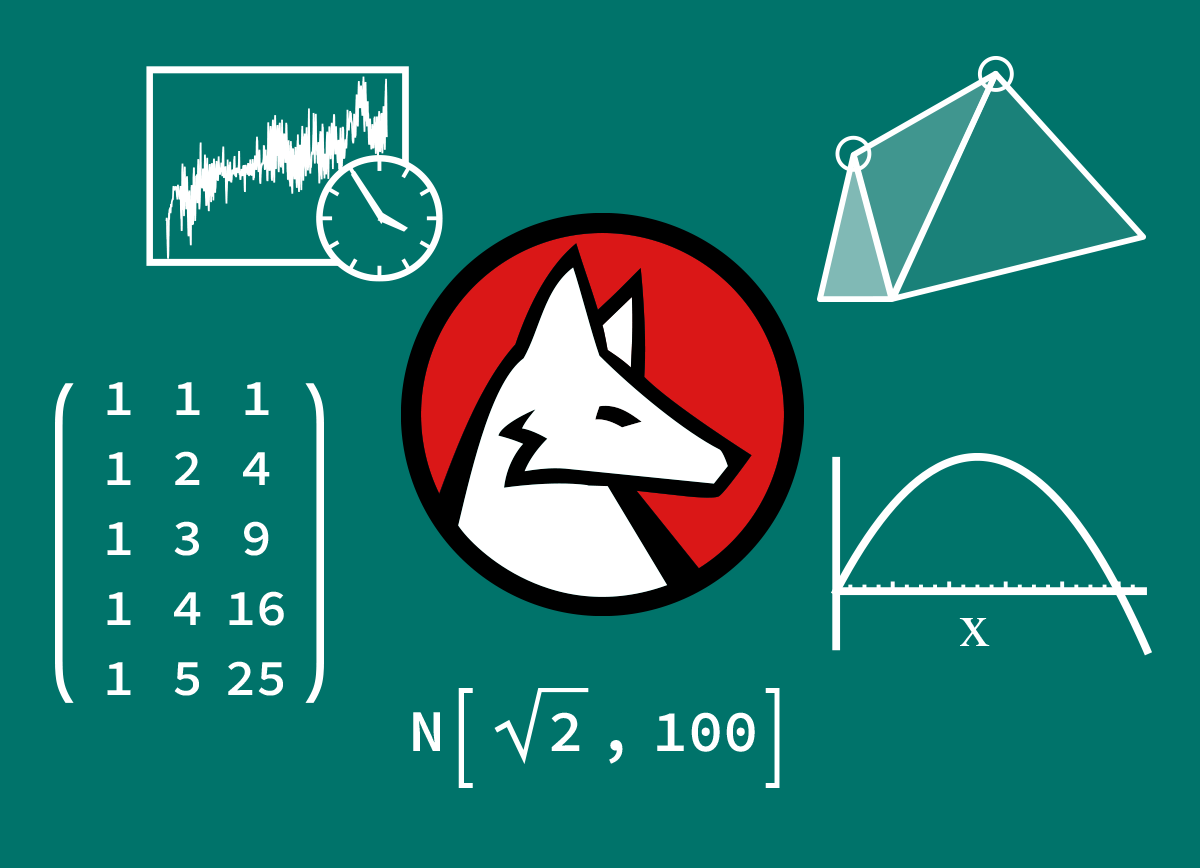##### A Guide to Mathematics with Wolfram Language
###### Video Course | FREE

Learn about using Wolfram Language for a broad range of mathematics. You can do symbolic, numeric and algebraic calculations, as well as geometry and calculus. These videos will introduce tools for solving and manipulating equations and calculations, including logical, Boolean and geometric expressions. Various built-in functions to help you solve polynomial and linear equations as well as number precision and order of interpolation are discussed. The videos cover common applied mathematics topics, such as matrices, linear algebra, optimization and numerical calculus. More advanced applications like time series calculations, image processing functionality and some data management are featured in later videos.

###### Course Overview
• Video 110 minutes
• Video 27 minutes
• Video 37 minutes
• Video 413 minutes
• Video 510 minutes
• Video 67 minutes
• Video 79 minutes
• Video 87 minutes
• Video 910 minutes
• Video 108 minutes
• Video 1110 minutes

Asymptotics: The Art of Approximation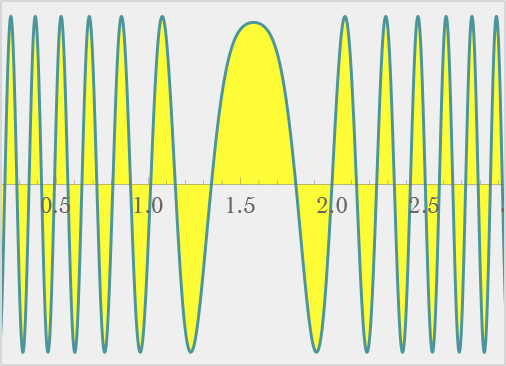##### Asymptotics: The Art of Approximation
###### Video Lesson | FREE

This video class introduces asymptotics as an alternative to exact and numerical methods. The class opens with some historical notes and explains how asymptotic methods can be useful. Examples shown include applications for the solution of differential equations, integrals, difference and algebraic equations and the computation of sums of infinite series. Learn how to use the built-in asymptotic functions in the Wolfram Language to solve hard problems that cannot be solved exactly.

Bringing Math to Life through Visual Learning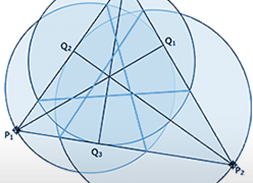##### Bringing Math to Life through Visual Learning
###### Video Lesson | FREE

This video class demonstrates how to encourage critical thinking in the classroom with the use of Wolfram|Alpha and the Wolfram Demonstrations Project. No previous experience with Mathematica or the Wolfram Language is required.

Calculus Applications and Algorithms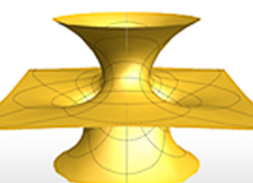##### Calculus Applications and Algorithms
###### Video Lesson | FREE

This class provides applications of calculus functions in the Wolfram Language and a discussion of the internal methods used for solving problems.

Calculus Fundamentals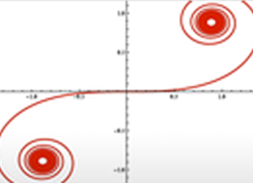##### Calculus Fundamentals
###### Video Lesson | FREE

This class gives a broad overview of calculus operations in the Wolfram Language. Learn details about the functionality for computing integrals and solving differential equations, and become familiar with the Wolfram Language built-in functions Integrate and DSolve. See how animations and interactive graphics are useful for understanding concepts. This class is suitable for beginners wanting to use Mathematica and the Wolfram Language to solve calculus problems.

Cryptography in Wolfram Language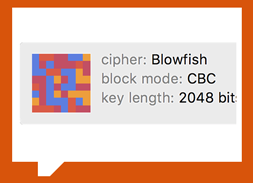##### Cryptography in Wolfram Language
###### Archived Event | FREE

In this recorded, two-part livecoding series, Christopher Wolfram discusses some basic concepts of cryptography and shows how they are implemented as built-in functions in Wolfram Language. Encryption examples are given using both symmetric (private-key) and asymmetric (public-key) cryptography. You'll learn how symmetric and asymmetric keys differ from each other and the contexts in which one is preferred over the other. The use of different ciphers and hash code types is shown, with discussion of password and digital signature encryption use cases.

###### Course Overview
• Video 19 minutes
• Video 263 minutes

Discrete Calculus Concepts and Applications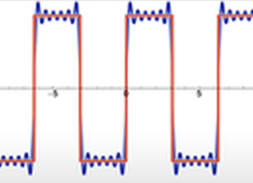##### Discrete Calculus Concepts and Applications
###### Video Lesson | FREE

This video gives a broad overview of discrete calculus functions in the Wolfram Language, along with applications to fractals, sequence recognition and the study of algorithms. This class requires no previous Wolfram Language experience.

Graphs and Networks: Concepts and Applications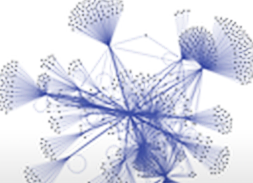##### Graphs and Networks: Concepts and Applications
###### Video Lesson | FREE

Graph theory and network analysis are used to model and analyze connections between entities in the real world—the World Wide Web, social networks and airline routes, just to name a few. The Wolfram Language provides a unified computational perspective from across multiple disciplines in the application of graphs and networks. This video class covers the fundamental properties of graphs and explores workflows available for network modeling and analysis.

## Course Type

• Interactive Courses
• Video Lessons
• Video Courses
• Instructor-led Courses
• Archived and Special Events

### Interactive Courses

Also known as MOOCs (massive open online courses), these courses are hosted on the Wolfram Cloud and allow you to interactively explore concepts using Wolfram Language functionality.

• Self-paced with progress tracking

• Include video lessons, exercises and problems, quizzes, exams and a scratch notebook

• Sharable completion certificates available for all courses

• Wolfram Level 1 proficiency certifications available for select courses

### Video Lessons

Short recorded lessons that provide limited instruction on a computational topic or for using Wolfram tech.

• Quick-start videos

• Lessons from content experts

• A wide variety of beginner-level lessons

• Free to watch

### Video Courses

Video series that build on preceding lessons to provide comprehensive instruction.

• Each video course features a playlist of sequential lessons

• Recorded by Wolfram certified instructors

• Comprehensive coverage of a particular topic

• Free to watch

### Instructor-led Courses

Scheduled as online and in-person classes, these courses provide comprehensive instruction guided by a live instructor.

• Registration required to reserve your seat

• Taught by Wolfram certified instructors

• Opportunity to pose live questions to experts in the room

• Course completion certificates available

### Archived and Special Events

Presentations by Wolfram developers, content experts and instructors.

• Webinars on special topics and new release functionality

• Livecoding sessions

• Wolfram Daily Study Groups

• Free to watch